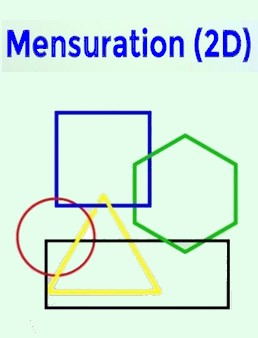# Mensuration 2D

Mensuration 2D is one of the important topics of Mathematics and forms the basis of other topics as well. This topic has a wide range of concepts for students to learn. Enroll for the ‘Mensuration 2D’ Course and learn all the concepts and valuable time saving tricks to solve Questions in No Time. Moreover, a wide range of Practice and Test Quizzes are also available in this Course through which you can Excel your problem solving skills in this topic.

₹ 10.00
₹ 10.00
Last Update 03/28/2021 1 day 8 hours 47 minutes 1
Key Features :
• Validity 1 Year
• Includes Short tricks
• Strong -Week Areas Analysis
• Bi-lingual (हिंदी + English)
• As per Latest Syllabus 2021-22
• Speed, Accuracy Boosters
• Includes Revision Class
• 200+ Practice Questions
• 100+ Imp Previous Year

• Introduction and Different 2D Shapes
• Free preview
• Free preview
• Free preview
• Curved Shapes
• Regular Polygon
• Rectangle
• Rectangle Class 1
• Rectangle Class 2
• Rectangle Class 3
• Square with rectangle
• Circle With rectangle
• Rectangle Other Conditions Questions
• Practice Questions based on Rectangle Only
• Practice Questions based on Rectangle with Others
• Square
• Square and its Diagonals
• Square and its perimeter
• Square Percentage Effects
• Circle with Square Class 1
• Circle With Square Class 2
• Triangle With Square
• Practice Questions based on Square
• Square With Other Conditions
• Parallelogram - Concept and Tricks
• Parallelogram Question Session
• Rhombus - Concept and Tricks
• Rhombus Question Session - Class 1
• Rhombus Question Session - Class 2
• Trapezium - Concept and Tricks
• Trapezium Question Session
• Triangle with Trapezium
• Circle With Trapezium
• Polygon - Concept and Questions
• Practice Questions on Quadrilaterals & Polygons
• Triangle
• Triangle - Concept and Tricks
• Congruent Triangles
• Similarity of Triangle
• Medians in Triangles
• Triangle Question Session 1
• Triangle Question Session 2
• Circle with Triangle - Class 1
• Circle with Triangle - Class 2
• Circle with Triangle - Class 3
• Triangle with Other Conditions
• Practice Questions based on Triangle
• Practice Questions based on Triangle with Others
• Circle
• Circle - Concept and tricks
• Circle Question Session 1
• Circle Question Session 2
• Circle Question Session 3
• Square with Circle
• Triangle with Circle
• Semi Circle - Concept & Tricks with Question Session
• Practice Questions based on Circle
• Mensuration 2D - Practice Quizzes
• Quiz 1 - Easy Level Questions
• Quiz 2 - Moderate Level Questions
• Quiz 3 - Advanced Level Questions